# R Lists

In this tutorial you will learn about the R Lists and its application with practical example.

## R Lists

List is a basic data structure that can contain elements of different types. It is similar to vectors except it can contains mixed data i.e. numbers, strings, vectors and another list inside it.

Example:-

## Creating a List

A list can be created using list() function as following –

Example:-

Following is an example to create a list containing strings, numbers, vectors and a logical values.

Output:-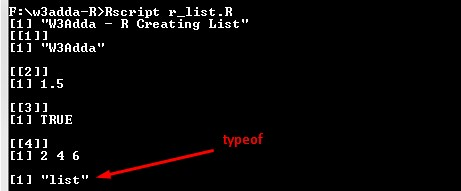## Naming List Elements

In R, we can assign name or tags to the list elements, that makes it easy to reference the individual element of the list.

Example:-

Following is an example to create same list with the tags as follows.

Here,

a, b and c are tags which makes it easier to reference the respective list element.

## Accessing List Elements

List elements can be accessed in same as of vectors. An index value can be logical, integer or character.

Example:-

Output:-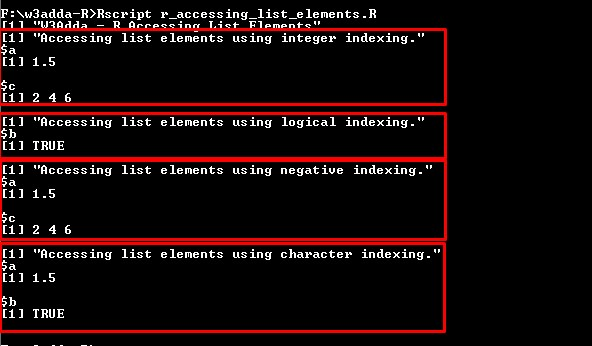An element can be simply added by assigning a values with new tags.

Example:-

Here, we have added a new list element with “d” tag and assigning it value “w3adda”. When we run the above R script, we will see following output –

Output:-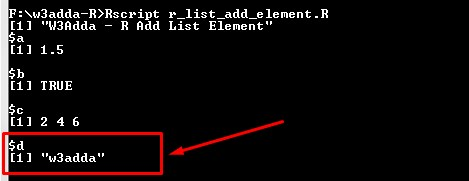## Update List Element

A list element can be modified by accessing element and assigning it new value.

Example:-

Here, we have updated list element with index position 1 with new value. When we run the above R script, we will see following output –

Output:-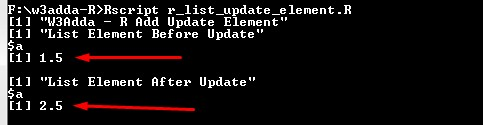## Delete Element From List

An element can be simply deleted by assigning NULL value to it.

Example:-

Here, we have deleted list element with index position 1 by assigning it NULL. When we run the above R script, we will see following output –

Output:-## Merging Lists

In R, we can create a new list by merging multiple list using list() function as following –

Example:-

Here, we have two list list1 and list2, and we have merge both of the list into list3. When we run the above R script, we will see following output –

Output:-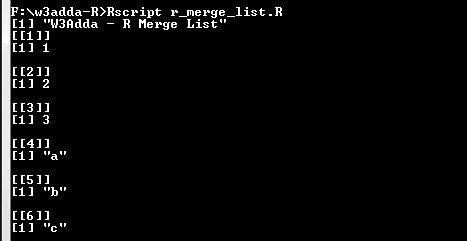## Converting List to Vector

The unlist() function can be used to convert a list object into a vector.

Example:-

Output:-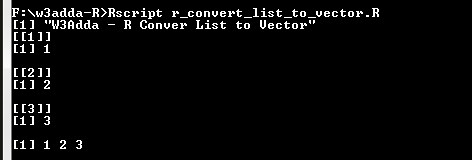In this tutorial we have learn about the R Lists and its application with practical example. I hope you will like this tutorial.